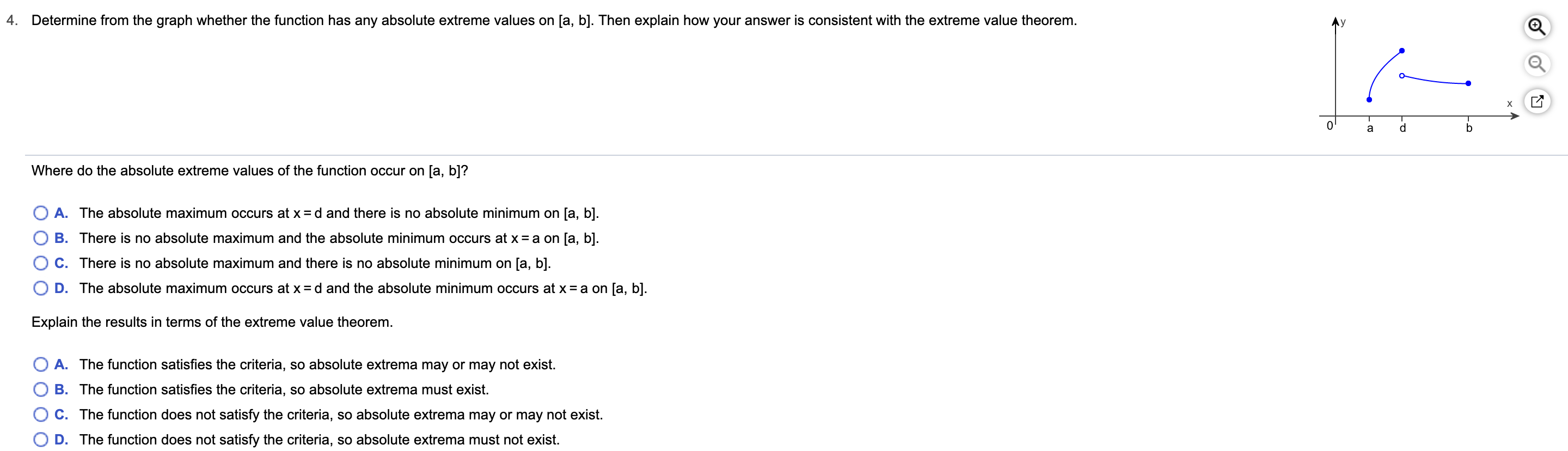# Determine from the graph whether the function has any absolute extreme values on [a, b]. Then explain how your answer is consistent with the extreme value theorem4.х0baWhere do the absolute extreme values of the function occur on [a, b]?A. The absolute maximum occurs at x =d and there is no absolute minimum on [a, b]B. There is no absolute maximum and the absolute minimum occurs at x = a on [a, b].C. There is no absolute maximum and there is no absolute minimum on [a, b].D. The absolute maximum occurs at x =d and the absolute minimum occurs at x = a on [a, b].Explain the results in terms of the extreme value theorem.A. The function satisfies the criteria, so absolute extrema may or may not exist.B. The function satisfies the criteria, so absolute extrema must exist.C. The function does not satisfy the criteria, so absolute extrema may or may not exist.D. The function does not satisfy the criteria, so absolute extrema must not exist.

Question

Can you help me step by step?help_outlineImage TranscriptioncloseDetermine from the graph whether the function has any absolute extreme values on [a, b]. Then explain how your answer is consistent with the extreme value theorem 4. х 0 b a Where do the absolute extreme values of the function occur on [a, b]? A. The absolute maximum occurs at x =d and there is no absolute minimum on [a, b] B. There is no absolute maximum and the absolute minimum occurs at x = a on [a, b]. C. There is no absolute maximum and there is no absolute minimum on [a, b]. D. The absolute maximum occurs at x =d and the absolute minimum occurs at x = a on [a, b]. Explain the results in terms of the extreme value theorem. A. The function satisfies the criteria, so absolute extrema may or may not exist. B. The function satisfies the criteria, so absolute extrema must exist. C. The function does not satisfy the criteria, so absolute extrema may or may not exist. D. The function does not satisfy the criteria, so absolute extrema must not exist. fullscreen
check_circle

Step 1

From the given graph it can be inferred that the absolute maximum is at x = d as y(d) is the maximum value.

Step 2

Similarly, the absolute minimum is at x = a as y(a...

### Want to see the full answer?

See Solution

#### Want to see this answer and more?

Solutions are written by subject experts who are available 24/7. Questions are typically answered within 1 hour.*

See Solution
*Response times may vary by subject and question.
Tagged in

### Calculus